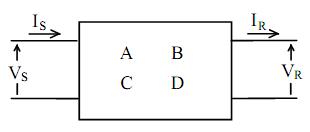## Explain how to represent power lines, Electrical Engineering

Assignment Help:

Explain How to Represent Power Lines?

The equivalent circuit of a power line depends on the length of the line and on the accuracy of the model required. In general, any power line may be represented as a two-port network, as shown in Figure, for which:

VS = AVR + B IR

IS = C VR + D IRThe line parameters used to determine the 2-port network constants (A, B, C and D) may be represented by lumped-constant circuits. For long transmission lines, a distributed-constant circuit model is used when accuracy is of importance.

#### Assignment, atomic absorption theory

atomic absorption theory

#### Give a block diagram for amodulo-5 binary ripple counter, Q. Give a block d...

Q. Give a block diagram for amodulo-5 binary ripple counter using JKFFs and draw its timing diagram.

#### Determine the laplace transform of the given functions, Q. Determine the La...

Q. Determine the Laplace transform for each of the following functions from the basic de?nition of Equation. (a) f 1 (t) = u(t) (b) f 2 (t) = e-at (c) f 3 (t) = df (t) dt,

#### Four quadrant chopper or class e chopper , Four  Quadrant Chopper or Class...

Four  Quadrant Chopper or Class E chopper                                                                    Figure class E chopper circuit  Mode I When

#### Digital control system, block diagram of digital control system and explain...

block diagram of digital control system and explain each block

#### What effect do non-tradable goods have on ppp, Q. What effect do non-tradab...

Q. What effect do non-tradable goods have on PPP? Answer: The consequence is quite substantial. In 1997 the production of non-tradable goods accounted for about 55% of U.S GN

#### Define number systems, Q. Define Number Systems? A number system, in ge...

Q. Define Number Systems? A number system, in general, is an ordered set of symbols (digits) with relationships defined for addition, subtraction, multiplication, and division.

#### Determine the voltage at the load terminals, Two single-phase 60-Hz sinusoi...

Two single-phase 60-Hz sinusoidal-source generators (with negligible internal impedances) are supplying to a common load of 10 kW at 0.8 power factor lagging. The impedance of the

#### Competition, how to be prepare for gate....

how to be prepare for gate....

#### RC coupled transistor amplifier., why we plot graph to find bandwidth is dr...

why we plot graph to find bandwidth is drawn b/w av/avmax v/s frequency not av v/s frequency?